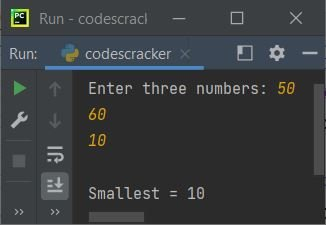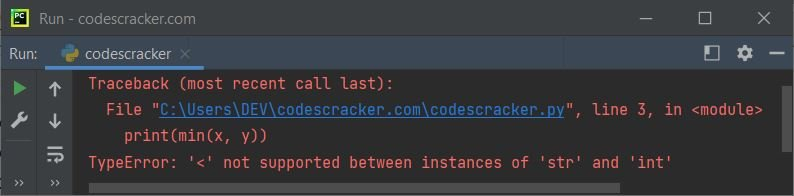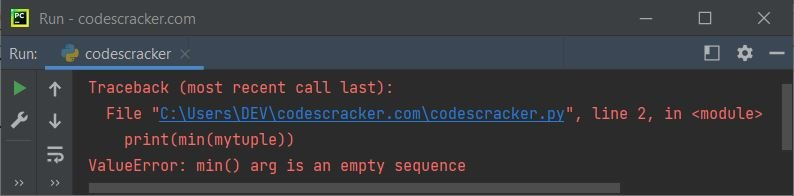# Python min() Function

The min() function in Python is used to find the smallest or minimum value among given set of values as its argument. This function can also be used to find the minimum item in an iterable. For example:

```print(min(12, 43, 6, 45))

mylist = [32, 54, 34, 13, 54, 65]
print(min(mylist))```

The output produced by above Python program, demonstrating the min() function is given below:

```6
13```

That is, 6 is the smallest number among 12, 43, 6, and 45. Whereas 13 is the smallest element among 32, 54, 34, 13, 54, and 65.

Note: The min() function does the similar job to max(). The only difference is that the min() returns smallest, whereas the max() returns the largest. That's it.

## Python min() Function Syntax

The syntax to use min() function in Python is:

`min(arg1, arg2, arg3, ..., argN, key)`

where arg1, arg2, and so on are values. The key parameter is used when we need to find the smallest string based on length, because the min() function, by default, returns the smallest string based on alphabetical order.

Note: Don't think much about the syntax, everything will get clear, using example programs given below.

Here is another syntax of min() function, when working with iterable.

`min(iter1, iter2, iter3, ..., iterN, key, default)`

Similar to previous syntax, iter1, iter2, and so on are iterable. The default parameter is used when we need to print the default minimum value, when the iterable is empty.

## Python min() Function Example

```print("Enter three numbers: ", end="")
x = int(input())
y = int(input())
z = int(input())

print("\nSmallest =", min(x, y, z))```

Sample run of this program, with user input 50, 60, 10 is shown in the snapshot given below:Now let's create another program that does the similar job as of above program. The only difference is, this program uses list to find the smallest element in the list. Since the program uses list, therefore I've created the program in a way to allow user to define the size of list too, along with its elements:

```print("Enter the value of n: ", end="")
n = int(input())
print("Enter", n, "numbers: ", end="")
mylist = []
for i in range(n):
num = int(input())
mylist.append(num)

print("\nSmallest =", min(mylist))```

Here is its sample run with user input 6 as size of list, 12, 23, 34, 45, 56, 67 as its six elements:Point to be Noted - If we pass strings as arguments of min() to find the minimum or smallest string, the function returns the smallest based on alphabetical order. For example:

```minstr = min("William", "Matthew", "Noah", "Ethan")
print(minstr)```

Following is the output produced by this Python program:

`Ethan`

based on alphabets, Ethan is the smallest one. Now what if we need to find the smallest string based on the number of character or length ?
Then we need to use the key parameter of min() function in that case. Here is an example:

```minstr = min("William", "Matthew", "Noah", "Ethan", key=len)
print(minstr)```

Now the output will be:

`Noah`

Here is another example demonstrates the min() function in Python:

```x = [12.4, 54.6, 1.43]
print(min(x))
print(min(12.32, 43, 54.65, 98.56, 32))
x = "codescracker"
print(min(x))
x = {"Name": "Daniel", "Region": "Hawaii"}
print(min(x))```

Following is the output of this program:

```1.43
12.32
a
Name```

### Handle Exception Raised by min() Function in Python

If we provide values of multiple types, then the min() function raises an exception named TypeError. For example, the following program:

```x = 23
y = "codescracker"
print(min(x, y))```

produces:Now to handle this exception, we need to wrap the min() inside a try block, so that the exception raised by this function can easily be caught using the except block, as shown in the program given below:

```x = 23
y = "codescracker"

try:
print(min(x, y))
except TypeError:
print("Invalid combination of arguments!")```

Now the output will be:

`Invalid combination of arguments!`

Of course the same program can also be created in this way:

```x = 23
y = "codescracker"

try:
smallest = min(x, y)
print(smallest)
except TypeError:
print("\nInvalid combination of arguments!")```

## The min() Function with Multiple Iterable

Here is an example of min() function with multiple iterable:

```x = [10, 4, 5, 6, 3, 32]
y = [32, 4, 6, 7]
z = [43, 6, 54, 76, 7]

print(min(x, y, z))```

Following is the output produced by this Python program:

`[10, 4, 5, 6, 3, 32]`

Since the first list, that is x has the smallest element, that is 3, among all the elements from all the lists, therefore the output is the first list, that is x.

### The min() Function with default Argument

This is the last program of this article, created to demonstrates, how the min() function with default argument is used:

```mylist = []
print(min(mylist, default=0))```

The output produced by this program will be 0. Because 0 is the default value that will be considered as the smallest one, in case if the iterable (list here) is empty. Basically the default parameter is used to avoid producing error, when the iterable is empty, or to consider the default value as the smallest, if the iterable is empty.

But if the iterable is empty, and the default parameter is not used, then the min() function produces an error. Let's take a look at the following program:

```mytuple = ()
print(min(mytuple))```

produces:Therefore either use the default parameter to avoid this error. But the problem is, what if we do not want to assign the default argument's value as the smallest, if the iterable is empty ?
Then in that case, you can put the min() function inside a try block to get the exception raised using the except block, as shown in the program given below:

```mytuple = ()
try:
print(min(mytuple))
except ValueError:
print("The tuple is empty!")```

now the output will be:

`The tuple is empty!`

Python Online Test

« Previous Function Next Function »